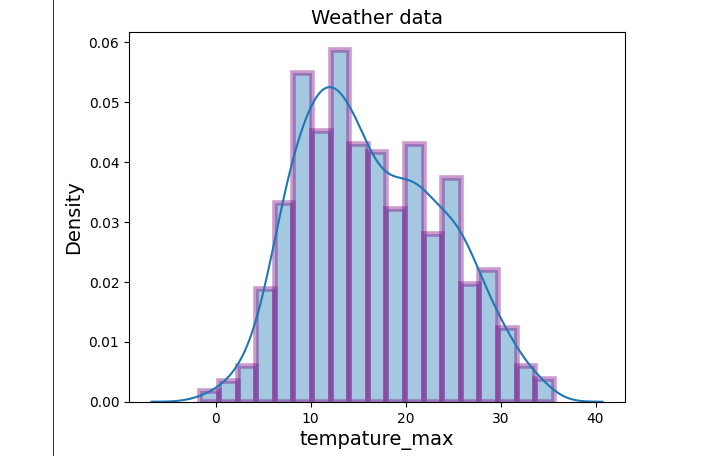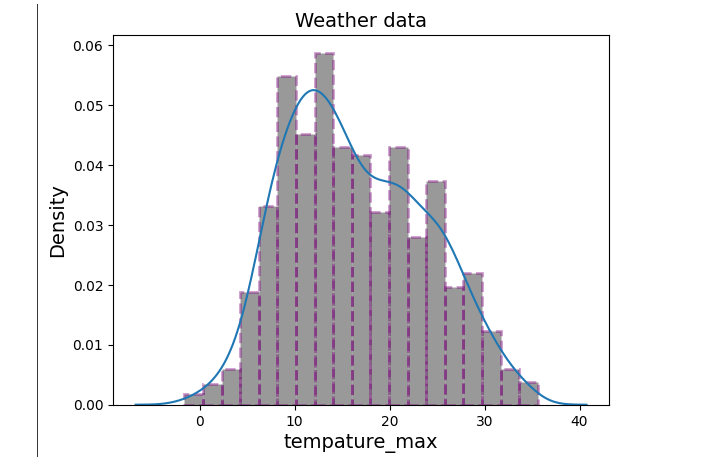# How to Add Outline or Edge Color to Histogram in Seaborn?

While Seaborn makes it easy to create histograms with a variety of styles and options, by default, histograms do not have an outline or edge color. Adding an outline or edge color can help make the plot more visually appealing and easier to interpret. In this article, we will explore how to add an outline or edge color to a histogram in Seaborn using a few simple steps. We will also discuss some of the customization options available to help create histograms that are tailored to your specific needs.

## What is a Histogram?

Histograms are used to show how one or more numerical variables are distributed. Seaborn helps us plot both the histogram bars and a density curve, which is made in the same way as kdeplots. To be precise, Histograms are graphical tools that show how a set of continuous data is distributed.

The histplot function in Seaborn is used to make histograms. The function can be called with the default values, which already make a pleasant chart. We can also manipulate ‘bins’ argument to change the number of bins. In fact, there can be a pattern hidden under the hood that you wouldn't be able to find with the default bins values.

In this article, we will add an outline or edge color to a histogram. This can be done using the function in seaborn which is seaborn.distplot() method/function.

## Seaborn

Seaborn is a Python library for making graphs based on statistics. It is built on top of matplotlib and works well with Pandas data structures.

Seaborn helps you look at your data and figure out what it means. Its plotting functions work on data frames and arrays that contain whole datasets and do the semantic mapping and statistical aggregation needed to make plots that are useful. Its declarative API is based on datasets, so you can focus on what the different parts of your plots mean instead of how to draw them.

Seaborn seeks to make visualizing data the primary method of looking at and understanding it. It gives us APIs that are focused on datasets, so we can switch between different ways of seeing the same variables to better understand the dataset.

We will use the seaborn.distplot() method in this article. Let’s discuss in detail the syntax for seaborn.distplot() method.

### seaborn.distplot()

A Distplot, also called a distribution plot, shows how the data are distributed. Seaborn Distplot shows how the continuous data variables are spread out.

With the help of the Seaborn module and the Matplotlib module, the distplot is shown in different ways. A histogram and a line are used together in the Distplot to show the data.

### Syntax

sns.distplot(aSeries)


Seaborn is usually imported under the sns alias.

Here aseries, list or one-dimensional array is the observed data. If this is a Series object with a name attribute, the label for the data axis will be the name.

### Parameters

S.No

Parameters and the their values

Definition

1

bins: argument for matplotlib hist() ,none, optional

Hist bin specifications

2

hist: bool, optional

It is used to specify whether we have to plot a normed histogram or not.

3

Kde: bool, optional

It is used to tell whether to plot Gaussian kernel density estimate or not

4

rug: bool, optional

It is used to specify whether to plot a rug plot on the support axis or not.

5

fit: object, optional

An object with a fit method that returns a tuple that can be passed as positional arguments to a pdf method to evaluate the pdf on a grid of values.

6

color: color, optional

Everything but the fitted curve should be colored in.

7

vertical: bool, optional

If the value of the parameter is true then the observed values are on the y-axis.

8

norm_hist: bool, optional

If True, the histogram height shows the number of items instead of how many there are. If a KDE or fitted density is shown, this is clear.

9

label: string, optional

Label the important part of the plot with a legend.

10

ax: axis, optional

If the value for this parameter is provided then plot on the axis provided.

## Program to Add Outline or Edge Color to Histogram in Seaborn

In this program, we will be using Seattle weather data from vega_datasets to build a histogram.

### Example

import seaborn as sns
import matplotlib.pyplot as plt
import numpy as npp
import pandas as pdd
from vega_datasets import data
sw= data.seattle_weather()
sns.distplot(sw['temp_max'], hist_kws=dict(edgecolor="purple", linewidth=4))
plt.title('Weather data', fontsize=14)
plt.xlabel('tempature_max', fontsize=14)
plt.ylabel('Density', fontsize=14)


### OutputIn this program, we will be using Seattle weather data from vega_datasets to build a histogram.

### Example

import seaborn as sns

import matplotlib.pyplot as pltt
import pandas as pdd
import numpy as npp
from vega_datasets import data
sw= data.la_riots()
sns.distplot(sw['age'],
hist_kws={'color':'pink', 'edgecolor':'green',
'linewidth':2, 'linestyle':'--'})
pltt.title('Sample data', fontsize=14)
pltt.xlabel('age', fontsize=14)


### Output## Conclusion

In this article, we have learned that a histogram depicts how continuous data is distributed and it is a visualization tool that comes under seaborn library. Seaborn is a Python library and it is used to construct a Histogram using seaborn.distplot() method. We have also constructed a histplot that has an outline or edge color by using the particular arguments in seaborn.distplot() method. We have used two different datasets (the Seattle weather dataset and la_riots) for constructing a histogram.

Updated on: 31-May-2023

247 Views